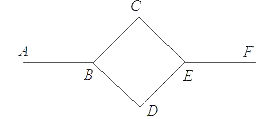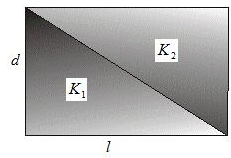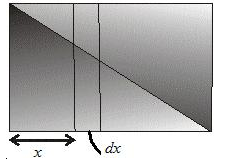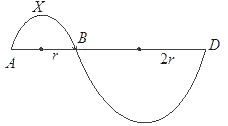# Heat transfer Problems

## Subjective Problems of Heat Transfer

Question 1
A rod is composed of two section whose length are $L_1$ and $L_2$ and heat conductivities are $K_1$ and $K_2$ respectively.One end of the rod is kept at $T_1$ and other end is kept at $T_2$.The rod is enclosed in a thermally insulating sheath.
a. Find the temperature of the interface.
b. Find the equivalent thermal conductivity of the system.
c. Find the heat current in the rod

Solution-1
Let T be the temperature of the interface.
Then in equilibrium
Heat flowing from left= heat flowing in the right
then
$K_1 \frac {(T_1-T)}{L_1}=K_2 \frac {(T-T_2)}{L_2}$
or $T= \frac {K_1T_1/L_1+K_2T_2/L_2}{K_1/L_1+K_2/L_2}$

Now
$K_1 \frac {(T_1-T)}{L_1}=K_2 \frac {(T-T_2)}{L_2} =K \frac {(T_1-T_2)}{L_1+L_2}$
or
$K= \frac {L_1+L_2}{L_1/K_1+L_2/K_2}$

Heat current.
$Q=K \frac {(T_1-T_2)}{L_1+L_2}$
$Q= \frac {T_1-T_2}{L_1/K_1+L_2/K_2}$

Question 2
An 1 mole of a gas whose adiabatic coefficient is 1.4 is at an initial temperature $T_0$ ,is enclosed in a cylindrical vessel fitted with a light piston. The surrounding temperature is $T_s$ and atmospheric pressure is $P_a$.Heat may be conducted between the surrounding and gas through the bottom of the cylinder. The bottom has surface area A, thickness is $L_1$ and thermal conductivity K.Assuming all the changes to be slow and ($T_s > T_0$).
a. find the temperature of the gas as a function of the time t.
b. Find the distance moved by the gas as function of the time t.

Heat will be transferred to the gas through the bottom of the cylinder and as the temperature of the gas increased, it will displace piston(Volume increase) since pressure is constant(atmospheric pressure)
Let x be the distance moved the piston at time t
Let T be the temperature of the gas at that moment.

Suppose an amount of heat dQ is transferred in time dt.

Then
$dQ=C_P dT$

Also $\frac {dQ}{dt}=\frac {KA(T_s-T)}{L_1}$

So
$C_P dT=\frac {KA(T_s-T)}{L_1}$ ----1

Also let $V_0$ is the initial volume of the gas.
Then $P_a V_0=RT_0$

or $V_0=\frac {RT_0}{P_a}$

Let V be the volume at time t
then
$\frac {V}{T}=\frac {V_0}{T_0}$ as Pressure remains constant throughout the process

Substituting V0 from last expression
then
$V= \frac {RT}{P_a}$
$V_0 + Ax= \frac {RT}{P_a}$
then

$\frac {RT_0}{P_a}+ Ax= \frac {RT}{P_a}$

$T=T_0 + \frac {P_aAx}{R}$

Differentiating both sides
$Adx=\frac {RdT}{P_a}$

Putting this is 1
$C_PAP_aL_1dx=KAR[T_s-T_0-(\frac {P_a Ax}{R})]dt$
$KRdt = \frac {C_P P_a L_1 dx}{T_s - T_0 - (P_a A x/R)}$
Integrating both sides with right side as upper and lower limit (t,0) and left side as upper and lower limit (x,0)
$\int_{0}^{t} KRdt = \int_{0}^{x} \frac {C_P P_a L_1 dx}{T_s - T_0 - (P_a A x/R)}$

$\frac {kAt}{L_1C_P}=ln[\frac {(T_s-T_0)}{(T_s-T_0-P_aAx/R)}]$
or $\frac {T_s-T_0}{T_s-T_0-P_aAx/R}= e^{kAt/L_1C_P}$
or $T_s-T_0-(P_aAx/R)=(T_s-T_0)e^{-kAt/L_1C_P}$
or
$x= \frac {R(T_s-T_0)(1-e^{-2kAt/7L_1R})}{P_a A}$

from ----1

$\frac {2KAdt}{7RL_1}=\frac {dT}{(T_s-T_0)}$

Integrating both sides with right side as upper and lower limit (t,0) and left side as upper and lower limit (T,$T_0$)

$ln \frac {T_s-T_0}{T_s-T}=\frac {2KAt}{7RL_1}$
or $T=T_s-(T_s-T_0)e^{-2KAt/7RL_1}$

Question 3
A body A of heat capacity s is kept in a surrounding where temperature is $T_a$.It cools down according Newton laws of cooling. The mass of the body is m and the body initial temperature is $T_0$.
a. Find the value of constant k if the temperature at time $t_1$ is $T_1$
b. finds the maximum heat body can lose.
c. Find the time at which body loses 80% of the total heat loss.
d. plots the graph for the logarithm of the numerical value of the temperature difference of the body A and its surrounding with respect to time t.

We have according to Newton laws of cooling.
$\frac {dT}{dt}=-k(T-T_a)$
$\frac {dT}{(T-T_a)}=-kdt$

Integrating both sides with right side as upper and lower limit ($T_1$,$T_0$) and left side as upper and lower limit ($t_1$,0)

$\int \frac {dT}{(T-T_a)}= \int -kdt$

$ln [\frac {(T_1-T_a)}{(T_0-T_a)}]=-kt_1$
$k = \frac {ln [(T_1-T_a)/(T_0-T_a)]}{t_1}$

The body continues to lose heat till it temperature becomes equal to surrounding.
So maximum heat body can lose

$\Delta Q_m=ms(T_0-T_a)$
Now if the body loses 80% of this heat in time $t_2$ and temperature is $T_{80}$
then
$.80 \Delta Q_m=ms(T_0-T_{80})$
$.8(T_0-T_a)=(T_0-T_{80})$

$T_{80}=.2T_0+.8T_a$.

Now again from Newton law of cooling
$\frac {dT}{(T-T_a)}=-kdt$

Integrating both sides with right side as upper and lower limit (T80,T0) and left side as upper and lower limit ($t_2$,0)

$\int \frac {dT}{(T-T_a)}=\int (-k)dt$
$ln \frac {(T_{80}-T_a)}{(T_0-T_a)}=-kt_2$

Substituting the value of k and T80
$t_2=ln( \frac {5}{k})$

Now
$T- T_a=(T_1-T_a)e^{-kt}$
Taking logarithm on both sides
$ln(T- T_a)=ln(T_1-T_a) -kT$

which is similarly to
y=mx+c

So graph will be a straight line.

Question 4
A rod of length l with thermally insulated lateral surface consists of a material whose heat conductivity coefficient varies with temperature given below
$K=\alpha T^{1/2}$ where α is constant.
The end of the rod are kept at the temperature $T_1$ and $T_2$ where $T_1 > T_2$.
Find the function T(x) where x is the distance from end where the temperature is $T_1$
And the heat flow through the rod

We have

Q=-αAT1/2dT/dx
Minus sign is there Temperature decreases with distance from the end $T_1$
Qdx=-αT1/2dT---1
Integrating both sides with right side as upper and lower limit (l,0) and left side as upper and lower limit ($T_2$,$T_1$)
∫Qdx=-∫αAT1/2dT
$Q=\frac {2 \alpha A(T_1^{3/2}-T_2^{3/2})}{3l}$

Now again Integrating equation 1 with right side as upper and lower limit (x,0) and left side as upper and lower limit (T,$T_1$)

∫Qdx=-∫αAT1/2dT
$Qx= \frac {2 \alpha A(T_1^{3/2}-T^{3/2})}{3}$

Substituting the value of Q from last expression
$T^{3/2}=T_1^{3/2} +( \frac {x}{l})(T_2^{3/2}-T_1^{3/2})$

or $T=T_1[1+(x/l)[(\frac {T_2}{T_1})^{3/2} -1]]^{2/3}]$

Question 5Six identical rods AB,BC,BD,CE,and DF are joined as shown in figure. The length ,cross-sectional area of each rod is A and L.
The thermal conductivities of the rods are as below
AB->K
BC and BD -> K/2
CE ,DE and EF ->K
The ends A and F are maintained at the temperature $T_1$ and $T_2$.Assuming no loss of heat to the atmosphere, find
a. Thermal resistance of the six rods
b. Thermal resistance of the whole system
c. The temperature at the points TB,TC,TD,TE
d. The heat flow ratio among the path BCE and BDE.

Thermal Resistance of Rod AB=(L/KA)
Thermal Resistance of Rod BC=(2L/KA)
Thermal Resistance of Rod BD=(2L/KA)
Thermal Resistance of Rod CE=(L/KA)
Thermal Resistance of Rod DE=(L/KA)
Thermal Resistance of Rod EF=(L/KA)

Now Rod BC and CE are in series So Total Thermal resistance
RBCE=RBC+RCE
=(3L/KA)

Similarly BD and DE are in series
then
RBDE=RBD+RDE
=(3L/KA)

Now Rod BCE and BDE are in parallel, so Net thermal resistance between ends B and E
(1/R)=(KA/3L)+(KA/3L)
R=(3L/2KA)

Now AB ,BE and EF are in series ,so net thermal resistance of the system is
R=(L/KA) + (3L/2KA) + (L/KA)
R=(7L/2KA)

So Net heat flow through the system
Q=(T_1-T_2)/R
Q=(2KA/7L)(T_1-T_2)

Now heat flow in Rod AB
Q=KA(TB -T_1)/L
Substituting the value of Q from equation 1

TB=(9T_1-2T_2)/7

Similarly for the heat flowing rod EF

Q=KA(TE -T_2)/L
Substituting the value of Q from equation 1
TE=(2T_1+5T_2)/7

Now Heat flow through rod BC =Heat flow through rod CE
(KA/2L)(TB-TC)=(KA/L)(TC-TE)
Substituting the values of TB and TE
we get
TC=(13T_1+8T_2)/21

Similarly the temperature at D
TD=(13T_1+8T_2)/21

Ratio of heat transfer =1:1

Question 6
A spherical shell has inner and outer radii as a and b respectively. And temperature at the inner and outer surface area are Ta and Tb And Ta > Tb.K is the thermal conductivity of the spherical shell.
Find the heat current through the shell

Let us draw two spherical shell with radii r and r+dr concentric with given system and Let T and T +dT be the temperature at them the heat flow through it

Q=-K(4πr2)(dT/dr)
-4πKdT=Q(dr/r2)

Integrating both sides with right side as lower and Upper limit (Ta,Tb) and left side as Lower and upper limit (a,b)

4πk(Ta-Tb)=Q[(1/a)-(1/b)]
$Q= \frac {4\pi kab(T_a-T_b)}{b-a}$

Question 7A rod of length l as shown is figure above with thermally insulated lateral surface is made of two material whose thermal conductivities are $K_1$ and $K_2$ respectively. The cross-sectional area is a square having edge d. Temperature at the ends are $T_1$ and $T_2$($T_1 > T_2$)
a, Find the heat flow
b. Equivalent thermal conductivity of the Rod

Considering a small portion of rod at distance x of thickness dx from the end whose temperature is T1Heat flow through the portion of the Rod is

Q=-K2(d2x/l)(dT/dx) -K1d(d -dx/l)(dT/dx)
as the edge at the portion at x for K2 is given by dx/l
-Q=dT/dx[K2(d2x/l) +K1d2 -K1(d2x/l)]
or
$-dT = \frac {Qdx}{K_2^2d^2x/l +K_1d^2 -K_1d^2x/l} Integrating both sides with right side as lower and Upper limit (T1,T2) and left side as lower and upper limit (0,l)$Q = \frac {d^2(T_1-T_2)(K_2-K_1)}{l[ln (K_2/K_1)]}$Now Q is also given by Q=Kd2(T1-T2)/l where K is the equivalent thermal conductivity of the system So comparing K=(K2-K1)/ln (K2/K1) Question 8 A cubical Block of mass 1 kg and edge 5 cm is heated to 227 °C.It is kept in a chamber which is maintained at the temperature 27 °C. Assuming the cubical block is having value of e=.5 a. Find the amount of radiation falling on the block. b. Find the net amount of heat flow outside the block. c. Find the rate at which temperature decrease at the beginning if specific heat=400J/kg-K d. if the chamber is maintained at the same temperature as the block. Find the heat absorbed and heat radiated by the block. σ=6.0 x 10-8 W/m2-K4 Answer Amount of the radiation by the block$=e \sigma AT_b^4$where Tb is body temperature. Substituting all the values Amount of the radiation by the block=28.12 W Amount of radiation absorb from the surrounding$=e \sigma AT_s^4$where Ts is surrounding temperature. Substituting all the values Amount of the radiation absorb from the surrounding =3.64 W Net heat flow outside the block=Amount of the radiation by the block - Amount of radiation absorb from the surrounding =24.38 Now$ms \frac {dT}{dt}=24.38 \frac {dT}{dt}=\frac {24.38}{ms}$=.06 °C/sec If the chamber is at the same temperature as the block. then Heat absorbed=28.12 W Heat radiated=28.12 W Question 9 One end of the rod of length L is inserted in furnace which is maintained at temperature$T_1$.The sides of the rod are covered with insulating material. Other end emits radiation with emissivity e. The temperature of the surrounding$T_s$.Let$T_2$is the temperature of the end in steady state. a. Find the thermal conductivity of the Rod b. Find the heat flow in the Rod. Answer Let k be the Thermal conductivity of the Rod. Then Heat flow through conduction in the Rod=Heat radiation from the Rod$ \frac {KA (T_1-T_2)}{L}=e \sigma A(T_2^4-T_s^4)$So$K= \frac {e \sigma L(T_2^4-T_s^4)}{(T_1-T_2)}$br /> Also$Q=e \sigma A(T_2^4-T_s^4)$Question 10 A Rod is initially at a uniform temperature at$T_1$.One end is kept at$T_1$and other end is kept in a furnace maintained at temperature at$T_2$.($T_2 > T_1$).The Surface of the rod is insulated so that heat can flow lengthwise along the rod.Lenght of the Rod is L, area A and thermal conductivity of the Rod is K.Consider a short cylindrical element of the rod of unit lenght.If the temperature gradient at the one end of the element is K'.Find the rate of flow across the element. Answer Q=KAK' Question 11 The tungsten element of the electric lamp has as surface area A and Power is P and emissivity is .4 a. Find the temperature of the filament b. if the tungsten filament behave like black-body ,find the % increase in power required to maintain the same temperature. Answer$P=e \sigma AT^4T=(\frac {P}{e \sigma A})^{1/4}$If the body behaves like black-body.$P_1= \sigma AT^4$Substituting the value of T from last expression$P_1= \frac {P}{e}$% increase in Power$ =\frac {P/e -P}{P} \times 100 = 150$% Question 12 A sphere, a cube and a circular plate have the same mass and are made of same material. All of them are at the same temperature T.Which one will have maximum rate of cooling. Answer For the same volume, Surface area of circular plate is maximum and Sphere is minimum. Now rate of cooling is$=e \sigma AT_2^4$So rate of cooling will be maximum for circular plate then the cube and it will be minimum for sphere. Question 13 A hole of radius$R_1$is made centrally in a circular disc of thickness d and radius$R_2$.The inner surface is maintained at temperature$T_1$and other surface is maintained at$T_2$($T_1 > T_2$).Thermal conductivity of the circular plate is K. a. Find the temperature as a function of radius from center b. Find the heat flow per unit time Answer$Q=-K \times 2 \pi r d \times (\frac {dT}{dr})\frac {Qdr}{r}=-2 \pi k d dT$Integrating both sides with right side as lower and Upper limit ($R_1$,$R_2$) and left side as lower and Upper limit ($T_1$,$T_2$)$\int \frac {Qdr}{r}= \int (-2 \pi kd)dTQ ln(\frac {R_2}{R_1})=2 \pi kd (T_1-T_2)$So$Q= \frac {2 \pi kd(T_1-T_2)}{ln(\frac {R_2}{R_1})}$Now Integrating (1) both sides with right side as lower and Upper limit ($R_1$,R) and left side as lower and upper limit ($T_1$,T)$\int \frac {Qdr}{r}= \int (-2 \pi kd)dTQ ln (\frac {R}{R_1})=2\ pi kd(T_1-T)$Substituting the value of Q$T=T_1 -(T_1 -T_2) \frac {ln (R/R_1)}{ln (R_2/R_1)}$Question 14 Two metallic sphere X & Y are made of same material and have identical surface finish. Let$m_1$,$m_2$are the masses of the spheres X & Y respectively. Both the sphere is at the same temperature. Both the sphere is placed in the same room having lower temperature. But they are thermally insulated from each other. Also$(\frac {m_1}{m_2})=n$. If the initial rate of cooling of sphere X to Y is (1/4)1/3.Find the value of n. Answer Rate of heat loss$ms \frac {dT}{dt}=4 \pi r^2 \sigma(T^4 - T_s^4)$Then Initial rate of cooling$\frac {dT}{dt}= \frac {4 \pi r^2 \sigma(T^4 - T_s^4)}{ms}$So$\frac {dT}{dt}$is proportional to$ \frac {r^2}{m}$For sphere X$(\frac {dT}{dt})_X= \frac {kr_1^2}{m_1}$For sphere Y$(\frac {dT}{dt})_Y= \frac {kr_2^2}{m_2}$Now let D be the density of the material$m_1=\frac {4}{3} \pi r_1^3 Dr_1=(\frac {3 \pi D m_1}{4})^{1/3}$Similarly$r_2=(\frac {3 \pi Dm_2}{4})^{1/3}$Ratio of initial rate of cooling$R= \frac {r_1^2 m_2}{r_2^2 m_1}R=\frac {m_1^{2/3} m_2}{m_2^{2/3} m_1}R= =(\frac {m_2}{m_1})^{1/3}=(\frac {1}{n})^{1/3}$Comparing from the given expression n=4 Question 15A system is shown in figure. It is made of semicircular Rod (AXB) which is joined at its ends to a straight rod AB of same material and the same cross-section .The straight Rod form a diameter of the other rod. Similarly semicircular Rod (BYD) which is joined at its ends to a straight rod of same material and cross-section area. a. Find the ratio of heat transfer across AXB and AB b. Find the ratio of heat transfer across BYD and BD Answer Ratio of heat transfer AXB and AB$=\frac { \frac {KA(T_a -T_b)}{\pi r}}{ \frac {KA(T_a-T_b)}{2r}}= \frac {2}{\pi} $Similarly for BYD and BD$=\frac {2}{\pi}\$